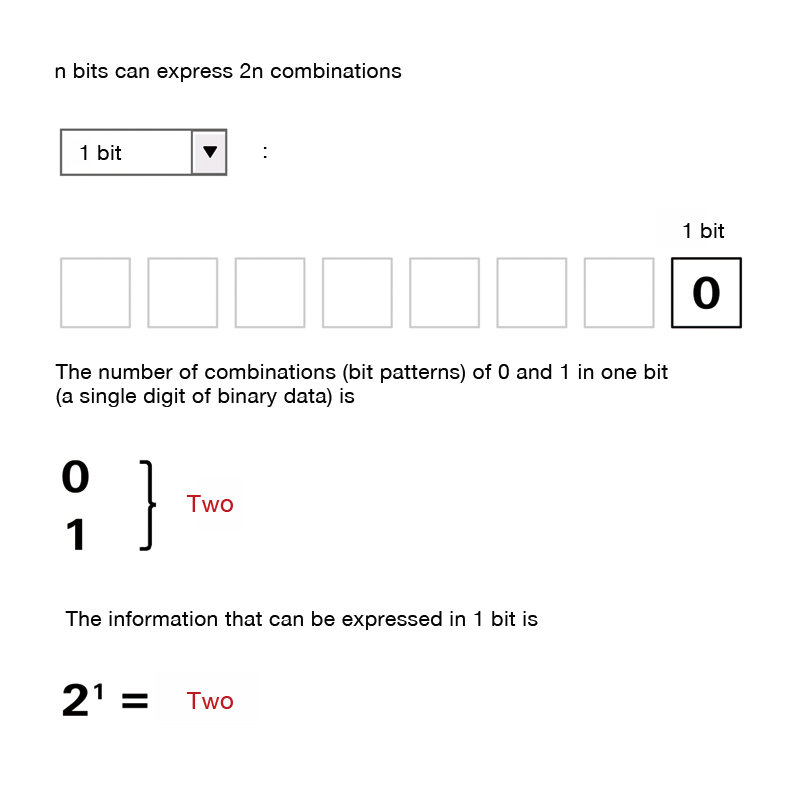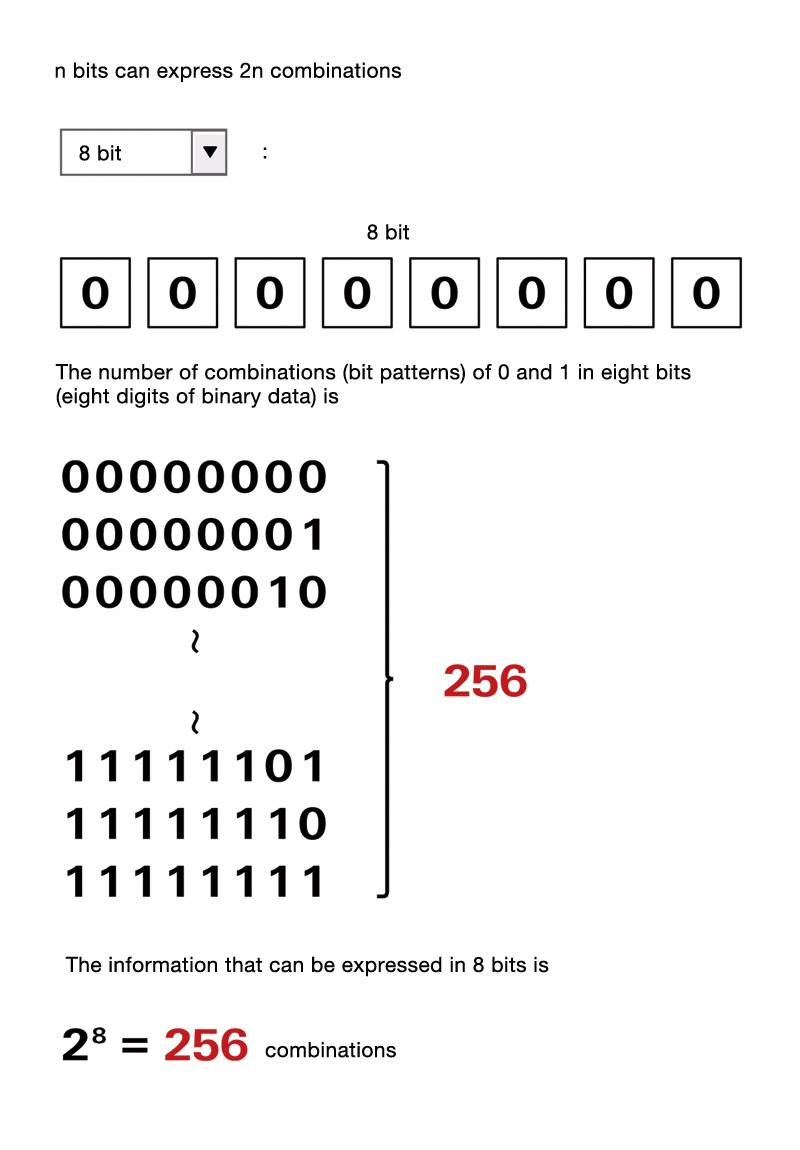# Bit

Bit

## CATEGORIES

When a computer processes information, it usually does so by expressing that information in 0s and 1s (binary digit expression). The smallest unit of that information (a single digit of binary data) is called a bit. This is the shortened version of the term, binary digit.

Digital data is founded on this idea of expressing information in 0s and 1s which can be represented in different ways depending on the medium. For example, with paper, digital data can be expressed by designating 0 and 1 according to whether or not there is a hole on it. Digital data can also be expressed using electrical charges, voltage and magnetism, so there are various recording media that utilize physical characteristics.

1 bit is the information that can be expressed using a single digit of binary data and it can express two states. The amount of information that can be expressed doubles each time the number of bits (digits) increases. Therefore, 2 bits can express 4 combinations and 3 bits 8 combinations, which means n bits can express 2 to the power of n combinations. If it is characters, 1 bit can express 2 characters, 2 bits 4 characters (22) and 8 bits 256 characters (28). If it is bitmap data colors, 1 bit can express 2 colors, 2 bits 4 colors and 8 bits 256 colors.

A unit called byte, consisting of 8 bits, is usually used to indicate the size of data on a computer. The CPU (Central Processing Unit) has a set number of bits it can process at a time. For example, a 32-bit CPU can handle data that is 32 bits long. The transmission speed of a network is expressed in bps (bits per second). This indicates how many bits of data can be transferred every second. So 10Mbps would mean that it is a network capable of transferring 10 megabits (10 million bits: 1.25 megabytes) of data in 1 second.

••